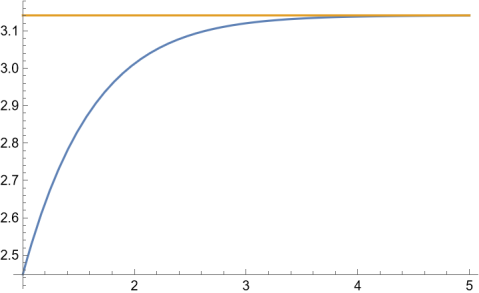# HAKMEM, AGM, and Pi

HAKMEM is a collection of tricks and trivia from the MIT AI lab written in 1972. I’ve mentioned HAKMEM here before. The image below came from HAKMEM item 123, explained here.I ran across HAKMEM item 143 while writing my previous two blog posts about the arithmetic-geometric mean (AGM). This entry, due to Gene Salamin, says thatfor large n. In fact, n doesn’t have to be very large. The expression on the right converges exponentially as n grows.Here’s the Mathematica code that produced the plot above.

    Plot[{2 n ArithmeticGeometricMean[1, 4 Exp[-n]], Pi},
{n, 1, 5}, PlotRange -> All]


If you have e to a large number of decimals, you could compute π efficiently by letting n be a power of 2, computing en by repeatedly squaring 1/e, and using the AGM. Computing the AGM is simple. In Python notation, iterate

    a, b = (a+b)/2, sqrt(a*b)

until a and b are equal modulo your tolerance.